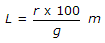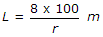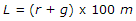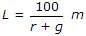# Civil Engineering - Highway Engineering

### Exercise :: Highway Engineering - Section 3

31.

If the velocity of moving vehicles on a road is 24 km/per hour, stopping distance is 19 metres and average length of vehicles is 6 metres, the basic capacity of lane, is

 A. 500 vehicles per hour B. 700 vehicles per hour C. 1000 vehicles per hour D. 1250 vehicles per hour

Explanation:

No answer description available for this question. Let us discuss.

32.

If the rate of change of grade permitted along a vertical curve is r and total change of grade is g%, the length L of the curve to be provided, is

 A.B.C.D.E. none of these.

Explanation:

No answer description available for this question. Let us discuss.

33.

In case of a multi-lane road, overtaking is generally permitted

 A. from right B. from left C. from both sides right and left D. not at all.

Explanation:

No answer description available for this question. Let us discuss.

34.

The pavement width of a road depends upon

 A. terrain B. type of traffic C. number of lanes D. all the above.

Explanation:

No answer description available for this question. Let us discuss.

35.

For a vehicle moving with a speed of 80 km per hour, the brake reaction time, in ordinary cases, is

 A. 1 sec B. 1.5 sec C. 2.0 sec D. 2.5 sec E. 3.0 sec